# cProfile Examples in Python

## Goal¶

This post aims to introduce how to use cProfile to measure the running time for each statement and find the bottleneck of your program.

## Libraries¶

In :
import cProfile


In :
def linear_func(n):
for i in range(n):
pass
return

for i in range(n):
for i in range(n):
pass

return

def exp_func(n):
if n <= 1:
return n
return exp_func(n-1) + exp_func(n-2)

In :
def main_func(n):
linear_func(n)
exp_func(n)


## Profile the main function¶

In :
cProfile.run('main_func(n=20)')

         21897 function calls (7 primitive calls) in 0.006 seconds

Ordered by: standard name

ncalls  tottime  percall  cumtime  percall filename:lineno(function)
1    0.000    0.000    0.000    0.000 <ipython-input-37-393400eb079b>:1(linear_func)
21891/1    0.006    0.000    0.006    0.006 <ipython-input-37-393400eb079b>:13(exp_func)
1    0.000    0.000    0.000    0.000 <ipython-input-37-393400eb079b>:6(quad_func)
1    0.000    0.000    0.006    0.006 <ipython-input-38-1333493d3326>:1(main_func)
1    0.000    0.000    0.006    0.006 <string>:1(<module>)
1    0.000    0.000    0.006    0.006 {built-in method builtins.exec}
1    0.000    0.000    0.000    0.000 {method 'disable' of '_lsprof.Profiler' objects}



## How to read the result¶

• ncalls is the number of calls.
• tottime is a total of the time spent.
• percall is the average time for each call, i.e., tottime divided by ncalls
• cumtime is the cumulative time spent.
• (2nd) percall is the quotient of cumtime divided by primitive calls
• filename:lineno(function) indicates the information about the function with the format "{file name}:{line number}{function name}"

# 104. Maximum Depth of Binary Tree

## Goal¶

This post aims to describe two solutions using recurrent function and queue for 104. Maximum Depth of Binary Tree.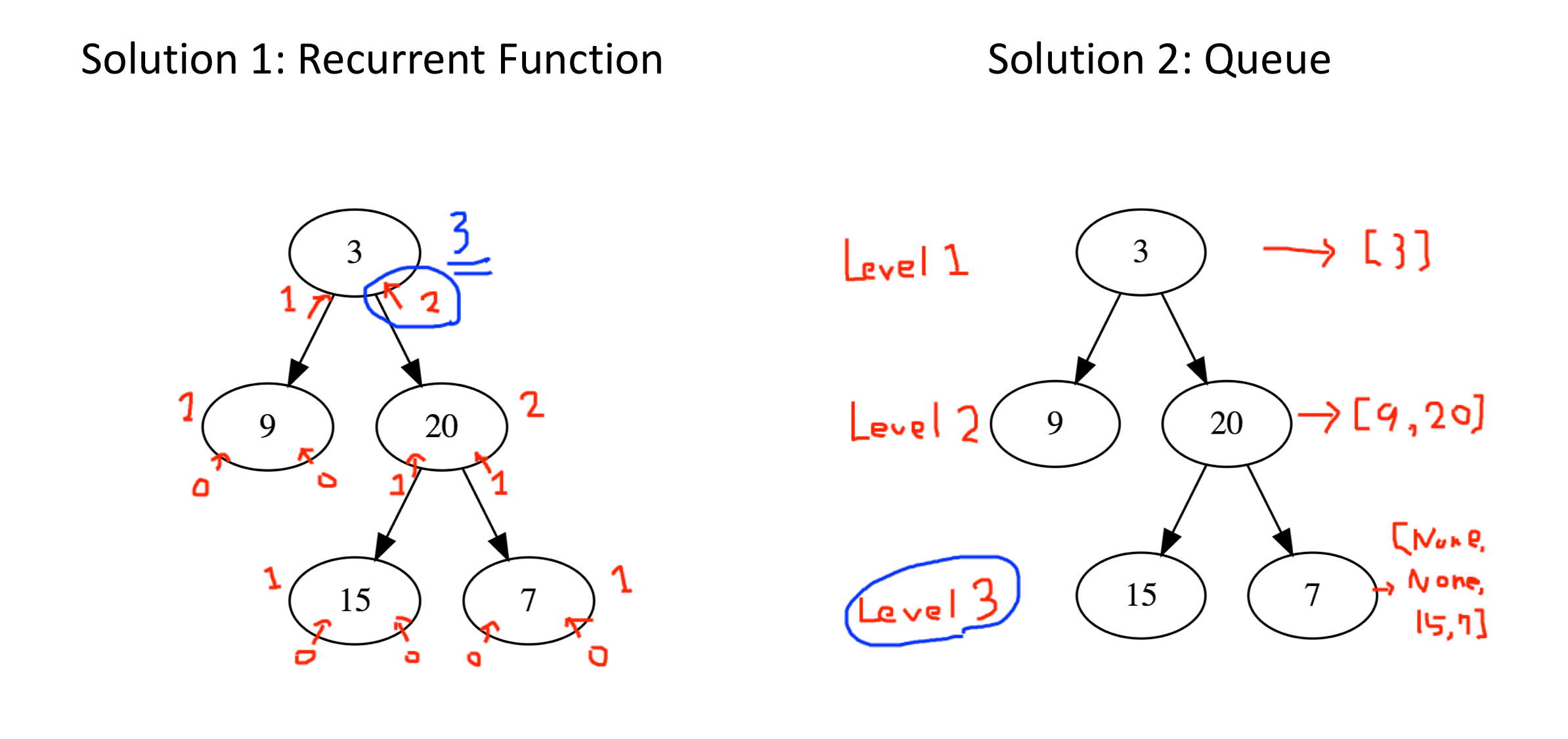# 1105. Filling Bookcase Shelves [Dynamic Programming]

## Goal¶

This post aims to describe the solution for 1105. Filling Bookcase Shelves based on the solution by a respectful coder Hexadecimal. This problem could be solved by dynamic programming.

## Problem¶

We have a sequence of books: the i-th book has thickness books[i] and height books[i].

We want to place these books in order onto bookcase shelves that have total width shelf_width.

We choose some of the books to place on this shelf (such that the sum of their thickness is <= shelf_width), then build another level of shelf of the bookcase so that the total height of the bookcase has increased by the maximum height of the books we just put down. We repeat this process until there are no more books to place.

Note again that at each step of the above process, the order of the books we place is the same order as the given sequence of books. For example, if we have an ordered list of 5 books, we might place the first and second book onto the first shelf, the third book on the second shelf, and the fourth and fifth book on the last shelf.

Return the minimum possible height that the total bookshelf can be after placing shelves in this manner.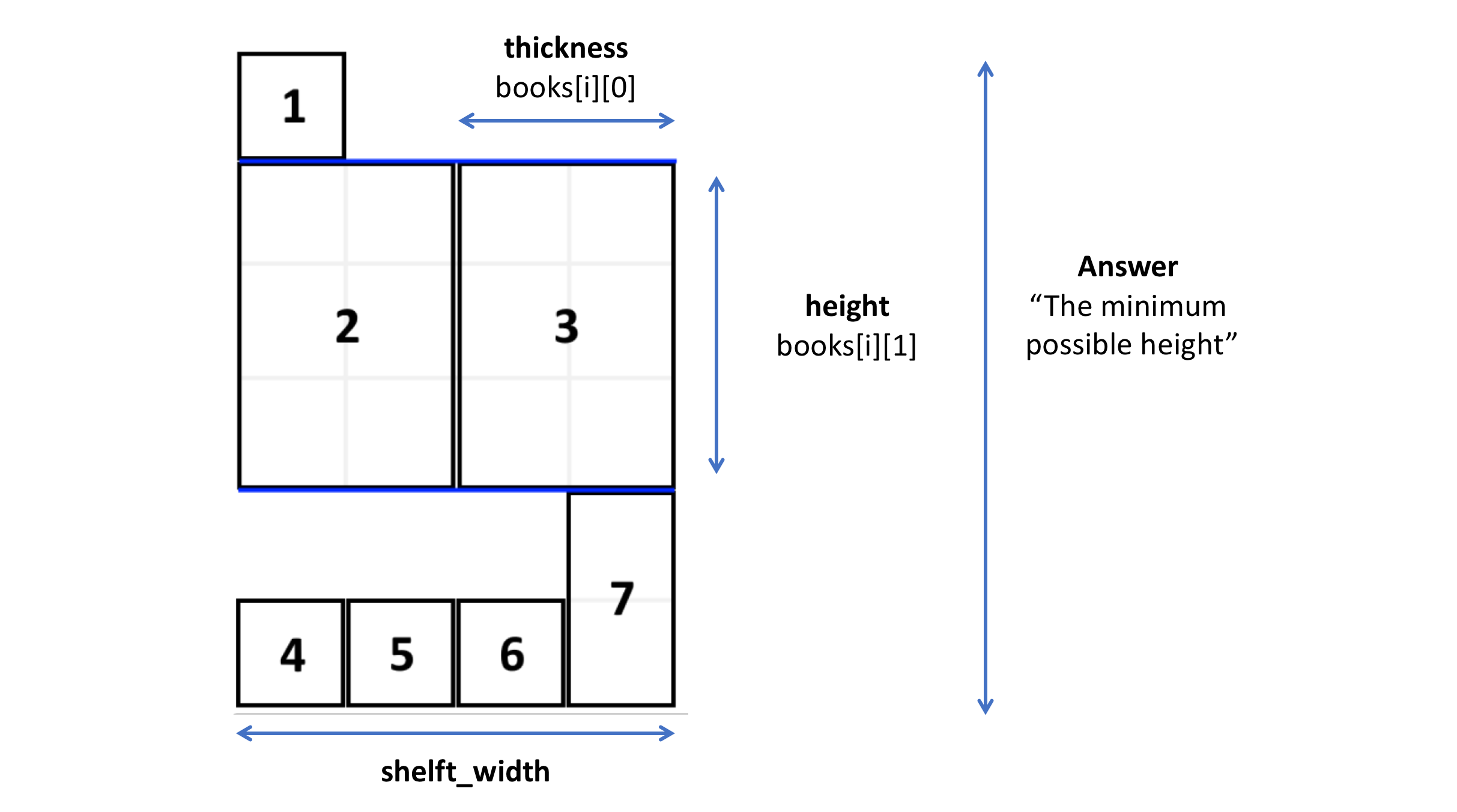# 1039. Minimum Score Triangulation of Polygon

## Problem Setting¶

Given N, consider a convex N-sided polygon with vertices labelled A, A[i], ..., A[N-1] in clockwise order.

Suppose you triangulate the polygon into N-2 triangles. For each triangle, the value of that triangle is the product of the labels of the vertices, and the total score of the triangulation is the sum of these values over all N-2 triangles in the triangulation.

Return the smallest possible total score that you can achieve with some triangulation of the polygon.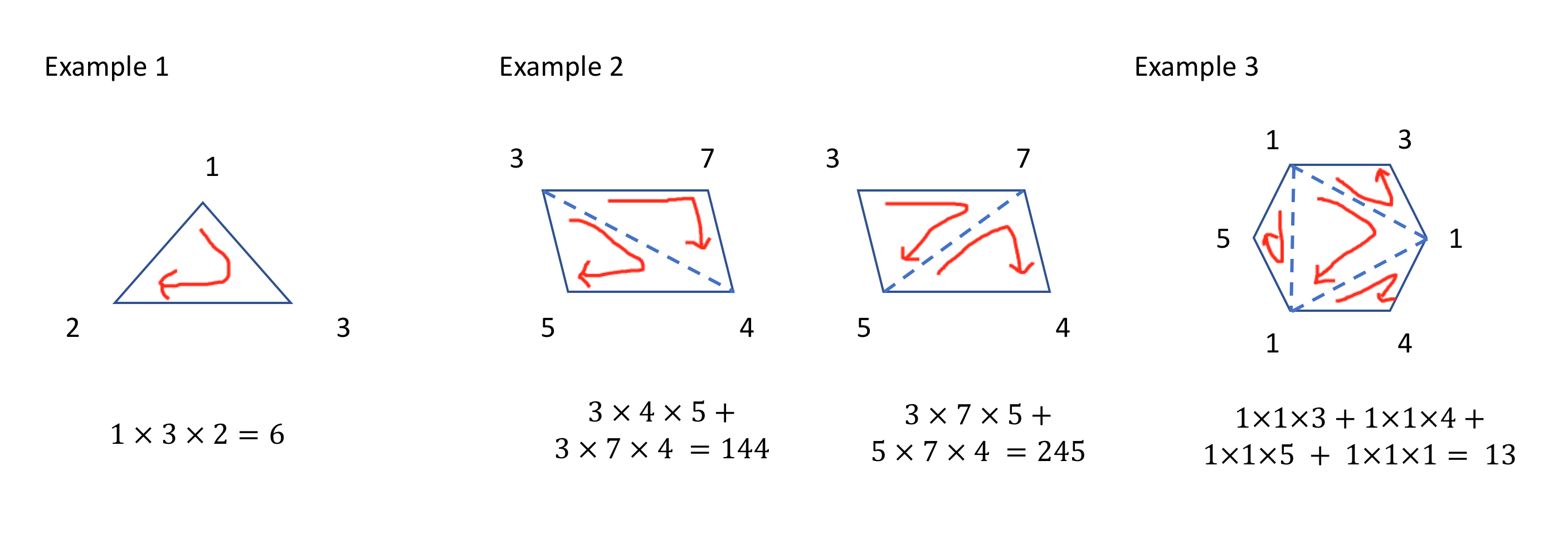The solution is based on dynamic programming like below. This gif is explained in the last part.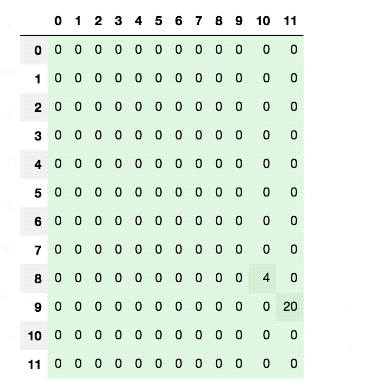# 1036. Escape a Large Maze

## Problem Setting¶

In a 1 million by 1 million grid, the coordinates of each grid square are (x, y) with 0 <= x, y < 10^6.

We start at the source square and want to reach the target square. Each move, we can walk to a 4-directionally adjacent square in the grid that isn't in the given list of blocked squares.

Return true if and only if it is possible to reach the target square through a sequence of moves

### Example 1:¶

Input: blocked = [[0,1],[1,0]], source = [0,0], target = [0,2]

Output: false

Explanation: The target square is inaccessible starting from the source square, because we can't walk outside the grid.

### Example 2:¶

Input: blocked = [], source = [0,0], target = [999999,999999]

Output: true

Explanation:

Because there are no blocked cells, it's possible to reach the target square.

# 1031. Maximum Sum of Two Non-Overlapping Subarrays

## Problem Setting¶

Given an array A of non-negative integers, return the maximum sum of elements in two non-overlapping (contiguous) subarrays, which have lengths L and M. (For clarification, the L-length subarray could occur before or after the M-length subarray.)

Formally, return the largest V for which V = (A[i] + A[i+1] + ... + A[i+L-1]) + (A[j] + A[j+1] + ... + A[j+M-1]) and either:

• 0 <= i < i + L - 1 < j < j + M - 1 < A.length, or
• 0 <= j < j + M - 1 < i < i + L - 1 < A.length.

### Example 1:¶

Input: A = [0,6,5,2,2,5,1,9,4], L = 1, M = 2

Output: 20

Explanation: One choice of subarrays is  with length 1, and [6,5] with length 2.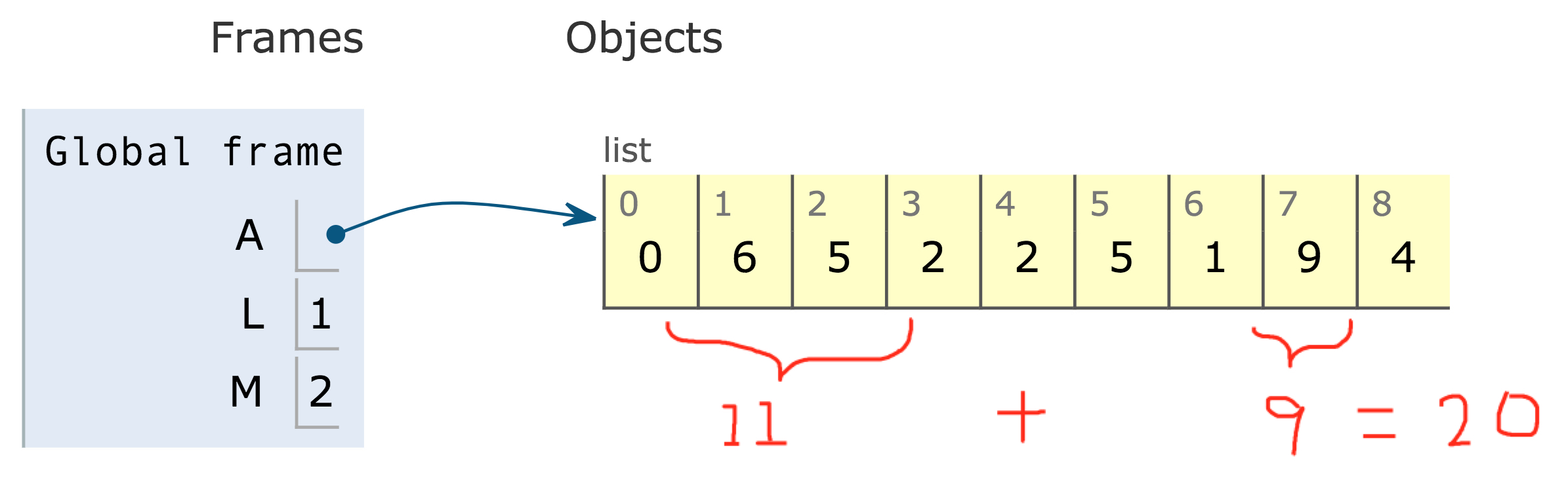# 1026. Maximum Difference Between Node and Ancestor

## Problem Setting¶

Given the root of a binary tree, find the maximum value V for which there exists different nodes A and B where V = |A.val - B.val| and A is an ancestor of B.

(A node A is an ancestor of B if either: any child of A is equal to B, or any child of A is an ancestor of B.)

Note:

• The number of nodes in the tree is between 2 and 5000.
• Each node will have value between 0 and 100000.

### Example 1¶

Input: [8,3,10,1,6,null,14,null,null,4,7,13]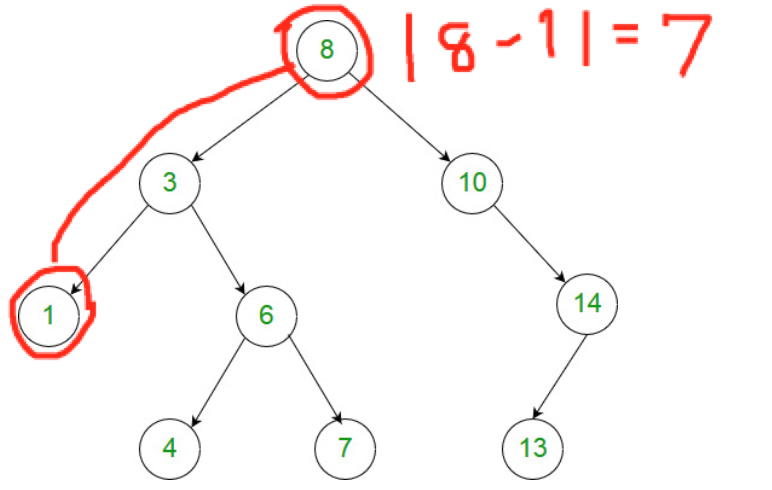Output: 7

Explanation:

We have various ancestor-node differences, some of which are given below : |8 - 3| = 5 |3 - 7| = 4 |8 - 1| = 7 |10 - 13| = 3 Among all possible differences, the maximum value of 7 is obtained by |8 - 1| = 7.

# LeetCode Top 100 Problem Selection

This link was posted on Dec 30, 2018 in blind Curated List of Top 100 LeetCode Questions. I found it so useful and would like to cover these problem in the following post as well.

Array

# 1025. Divisor Game (C++)

## Problem Setting¶

Alice and Bob take turns playing a game, with Alice starting first.

Initially, there is a number N on the chalkboard. On each player's turn, that player makes a move consisting of:

Choosing any x with 0 < x < N and N % x == 0. Replacing the number N on the chalkboard with N - x. Also, if a player cannot make a move, they lose the game.

Return True if and only if Alice wins the game, assuming both players play optimally.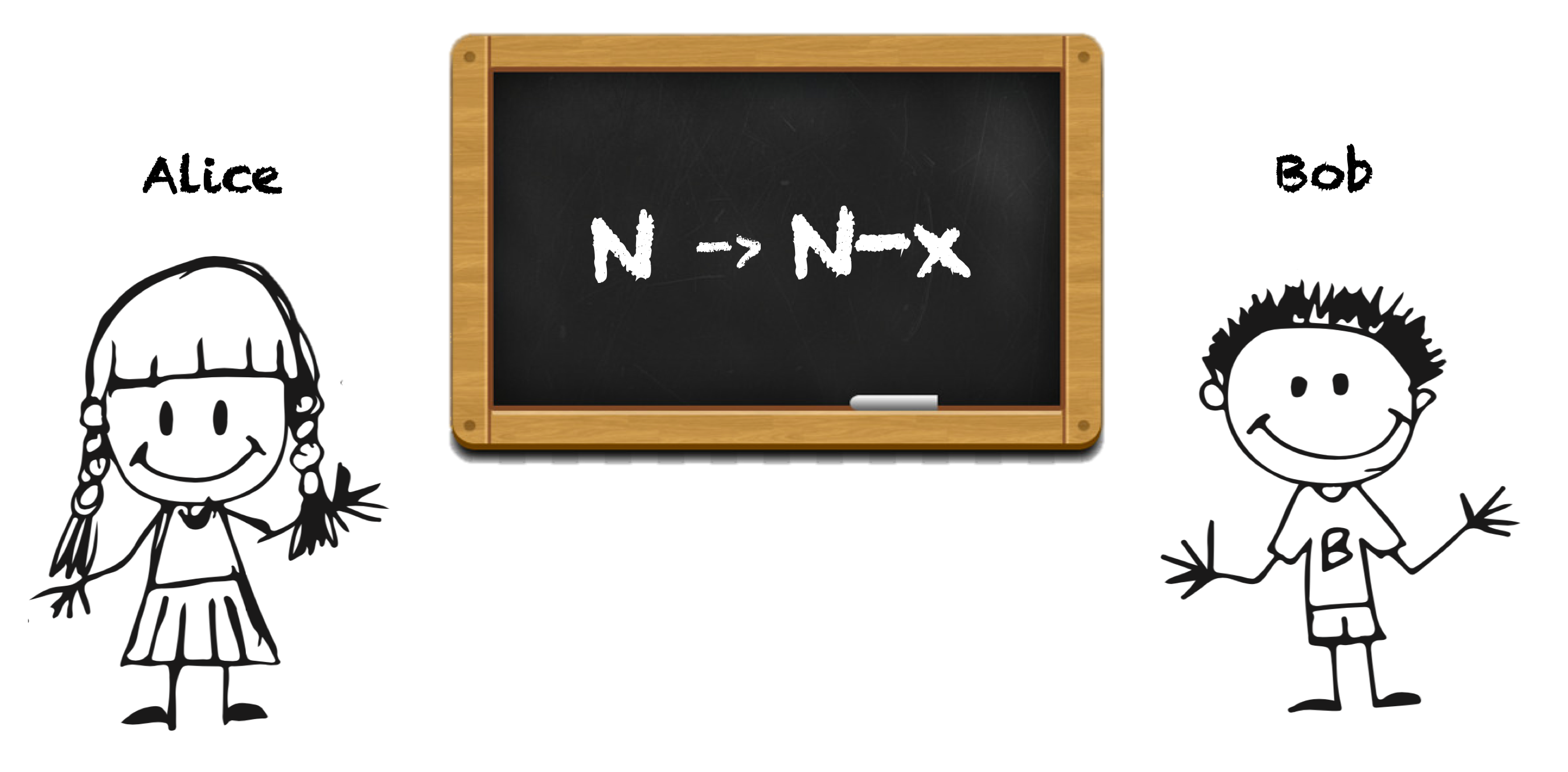# 1019. Next Greater Node In Linked List

## Problem Setting¶

We are given a linked list with head as the first node. Let's number the nodes in the list: node_1, node_2, node_3, ... etc.

Each node may have a next larger value: for node_i, next_larger(node_i) is the node_j.val such that j > i, node_j.val > node_i.val, and j is the smallest possible choice. If such a j does not exist, the next larger value is 0.

Return an array of integers answer, where answer[i] = next_larger(node_{i+1}).

Note that in the example inputs (not outputs) below, arrays such as [2,1,5] represent the serialization of a linked list with a head node value of 2, second node value of 1, and third node value of 5.

Variable Type Description
answer list of int an array of integers where answer[i] = next_larger(node_{i+1})
from graphviz import Digraph